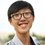# Function Challenge

For the function listed below, try expressing them in a single formula.

Example :

$f(x) = \begin{cases} -x & \quad \text{if } x \geq 0\\ x & \quad \text{if } x<0\\ \end{cases}$

Answer : $f(x) = -|x|$

Challenge 1 :

$f(x) = \begin{cases} 1 & \quad \text{if } x < 0\\ -1 & \quad \text{if } x>0\\ \end{cases}$

Challenge 2 :

$f(x) = \begin{cases} 0 & \quad \text{if } x\leq 0\\ x & \quad \text{if } x>0\\ \end{cases}$

Challenge 3 :

$f(x,y) = \begin{cases} x-y & \quad \text{if } x > y\\ x+y & \quad \text{if } x < y\\ \end{cases}$Note by Christopher Boo
5 years, 6 months ago

This discussion board is a place to discuss our Daily Challenges and the math and science related to those challenges. Explanations are more than just a solution — they should explain the steps and thinking strategies that you used to obtain the solution. Comments should further the discussion of math and science.

When posting on Brilliant:

• Use the emojis to react to an explanation, whether you're congratulating a job well done , or just really confused .
• Ask specific questions about the challenge or the steps in somebody's explanation. Well-posed questions can add a lot to the discussion, but posting "I don't understand!" doesn't help anyone.
• Try to contribute something new to the discussion, whether it is an extension, generalization or other idea related to the challenge.

MarkdownAppears as
*italics* or _italics_ italics
**bold** or __bold__ bold
- bulleted- list
• bulleted
• list
1. numbered2. list
1. numbered
2. list
Note: you must add a full line of space before and after lists for them to show up correctly
paragraph 1paragraph 2

paragraph 1

paragraph 2

[example link](https://brilliant.org)example link
> This is a quote
This is a quote
    # I indented these lines
# 4 spaces, and now they show
# up as a code block.

print "hello world"
# I indented these lines
# 4 spaces, and now they show
# up as a code block.

print "hello world"
MathAppears as
Remember to wrap math in $$ ... $$ or $ ... $ to ensure proper formatting.
2 \times 3 $2 \times 3$
2^{34} $2^{34}$
a_{i-1} $a_{i-1}$
\frac{2}{3} $\frac{2}{3}$
\sqrt{2} $\sqrt{2}$
\sum_{i=1}^3 $\sum_{i=1}^3$
\sin \theta $\sin \theta$
\boxed{123} $\boxed{123}$

Sort by:

1)$-\dfrac{x}{|x|}$ 2)$\left|\dfrac{x}{2}\right|+\dfrac{x}{2}$ 3)$\dfrac{(x-y)(x+y)}{\left(x+\dfrac{|x-y|y}{x-y}\right)}$

- 5 years, 6 months ago

1. $f_1(x)=-\frac {|x|}{x}$

2. $f_2(x)=\frac {|x|+x}{2}$

3. We can find a solution by using the solutions for challenge 1 and 2:

I don't know how to type arrays so let $1,2$ denote the conditions $y-x<0, y-x>0$ respectively.

Note that $f_2(y-x)+x=1.x, 2.y$ and $f_2(y-x)-y=1.-y, 2.-x$.

Further, $f_1(y-x)(f_2(y-x)-y)=1.-y, 2.x$

Hence $f(x)=f_2(y-x)+x+f_1(y-x)(f_2(y-x)-y)=1.x-y, 2.x+y$

Another way is by considering $\frac {1+f_1(y-x)}{2}=1.1, 2.0, \frac {1-f_1(y-x)}{2}=1.0, 2.1$.

Thus, $f(x)=(x-y)\frac {1+f_1(y-x)}{2}+(x+y)\frac {1-f_1(y-x)}{2}$

- 5 years, 6 months ago

For the first function, $f(x) = 1_{\{x|x<0\}} - 1_{\{x|x>0\}}$ where $f : \mathbb{R} \setminus \{0\} \to \mathbb{R}$. Because $1_S$ is the characteristic function that returns $1$ if the argument is in $S$ and $0$ otherwise.

If you want me to nitpick even more, those given aren't functions since you don't specify the domain (and the range, but the range usually can be inferred assuming it fits the whole domain). If I assume the domain of the first function is $\{1\}$, then my "formula" can be as simple as $f(x) = -1$ (because it only needs to work for the single number $x = 1$ in the domain).

A more interesting question is why you want to look specifically for just a single formula, and not just leave the function piecewise which is much easier to understand compared to the single formula forms?

- 5 years, 6 months ago

Compute the value of

$\sin 10^\circ \sin 30^\circ \sin 50^\circ \sin 70^\circ$

without using a calculator.

Why shouldn't we use a calculator when we can? Isn't using a calculator faster and is less likely to have errors?

- 5 years, 6 months ago

You can't use calculator there because the result is rational. A calculator might round/truncate the result midway. I did throw it to WolframAlpha anyway, though.

- 5 years, 6 months ago

@Ivan Koswara, imagine you are solving a hard equation. yo just spend a huge time on it and are feeling proud of what you did! You want to show other people this cool eqn but when you show this to yor classmates, who suck at maths, say "waste of time, i solved it in a second by putting it in wolfram alpha. i am smarter then you."

the "imagine" is taken from real life!

- 5 years, 6 months ago